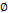Archive
Special Issues
Quadratic Optimal Control of Fractional Stochastic Differential Equation with Application
Science Journal of Applied Mathematics and Statistics
Volume 4, Issue 4, August 2016, Pages: 147-158
Received: May 4, 2016; Accepted: Jun. 3, 2016; Published: Jul. 23, 2016
Authors
Sameer Qasim Hasan, College of Education, Almustansryah University, Baghdad, Iraq
Gaeth Ali Salum, College of Education, Almustansryah University, Baghdad, Iraq
Article ToolsAbstractPDF (295KB)HTML
Abstract
The paper is devoted to the study of optimal control of Quadratic Optimal Control of Fractional stochastic differential Equation with application of Economy Mode with different types of fractional stochastic formula (ITO, Stratonovich), By using the Dynkin formula, Hamilton-Jacobi-Bellman (HJB) equation and the inverse HJB equation are derived. Application is given to a stochastic model in economics.
Keywords
Fractional Stochastic Differential Equations, Dynkine Formula, Hamilton-Jacobi-Bellman Equation
Sameer Qasim Hasan, Gaeth Ali Salum, Quadratic Optimal Control of Fractional Stochastic Differential Equation with Application, Science Journal of Applied Mathematics and Statistics. Vol. 4, No. 4, 2016, pp. 147-158. doi: 10.11648/j.sjams.20160404.15
References

A. F. Ivanov and A. V. Swishchuk, optimal control of stochastic differential delay equation. prepriat, December 2003, 6pp (Applied Math. Letters, sub-mitted).

B.ksendal, Stochastic Differential Equations. May 2000, Springer-verlag Berlin Heidelberg New York.

E. Allen, Modeling with ITO Stochastic Differential Equation. 2007-springer.

F. P. Ramsey, "Amathemutical theory of savings". Economic J. 388 (1928), 543-549.

Ganig., Heyde C. C., Jagers p. and Kurtz T. G., "probability and its Application", Springer-verlag London Limited, 2008.

G. Gandolfo, "Economic Dynamics", springer-verlag, 1996.

Javier R. Movellan, "Tutorial On Stochastic Differential Equation", 2011.

K. E. Peter, Numerical solution of Stochastic Differential Equation. 1990 (springer-verlag Berlin.

Nualart D., "Fractional Brounian Motion: stochastic calculus and Applications", proceeding Mathemati

T. E. Duncan and B. pasik-Duncan, An approach to stochastic Integration for Fractional Brownian Motion in a Hilbert space.
PUBLICATION SERVICES